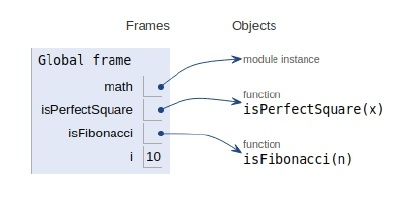# Python Program for How to check if a given number is a Fibonacci number?

## Problem statement

Given a number n, check whether n is a Fibonacci number or not

We all are aware that the nth Fibonacci number is the sum of the previous two Fibonacci numbers. But they also offer an interesting relation other than the recurrence relation.

A number is Fibonacci in nature if and only if (5*n2 + 4) or (5*n2 – 4) is a perfect square.

We will use this property to check whether a number is Fibonacci or not.

Now let’s see the implementation of the Python script −

## Example

Live Demo

import math
# if x is perfect square
def isPerfectSquare(x):
s = int(math.sqrt(x))
return s*s == x
# if n is a Fibinacci Number
def isFibonacci(n):
#if one of 5*n*n + 4 or 5*n*n - 4 or both is a perferct square
return isPerfectSquare(5*n*n + 4) or isPerfectSquare(5*n*n - 4)
for i in range(1,11):
if (isFibonacci(i) == True):
print (i,"is a Fibonacci Number")
else:
print (i,"is a not Fibonacci Number")

## Output

1 is a Fibonacci Number
2 is a Fibonacci Number
3 is a Fibonacci Number
4 is a not Fibonacci Number
5 is a Fibonacci Number
6 is a not Fibonacci Number
7 is a not Fibonacci Number
8 is a Fibonacci Number
9 is a not Fibonacci Number
10 is a not Fibonacci Number

All functions and variables are declared in the global frame as shown in the image below −## Conclusion

In this article, we learned the solution of identifying that the given number is Fibonacci or not.